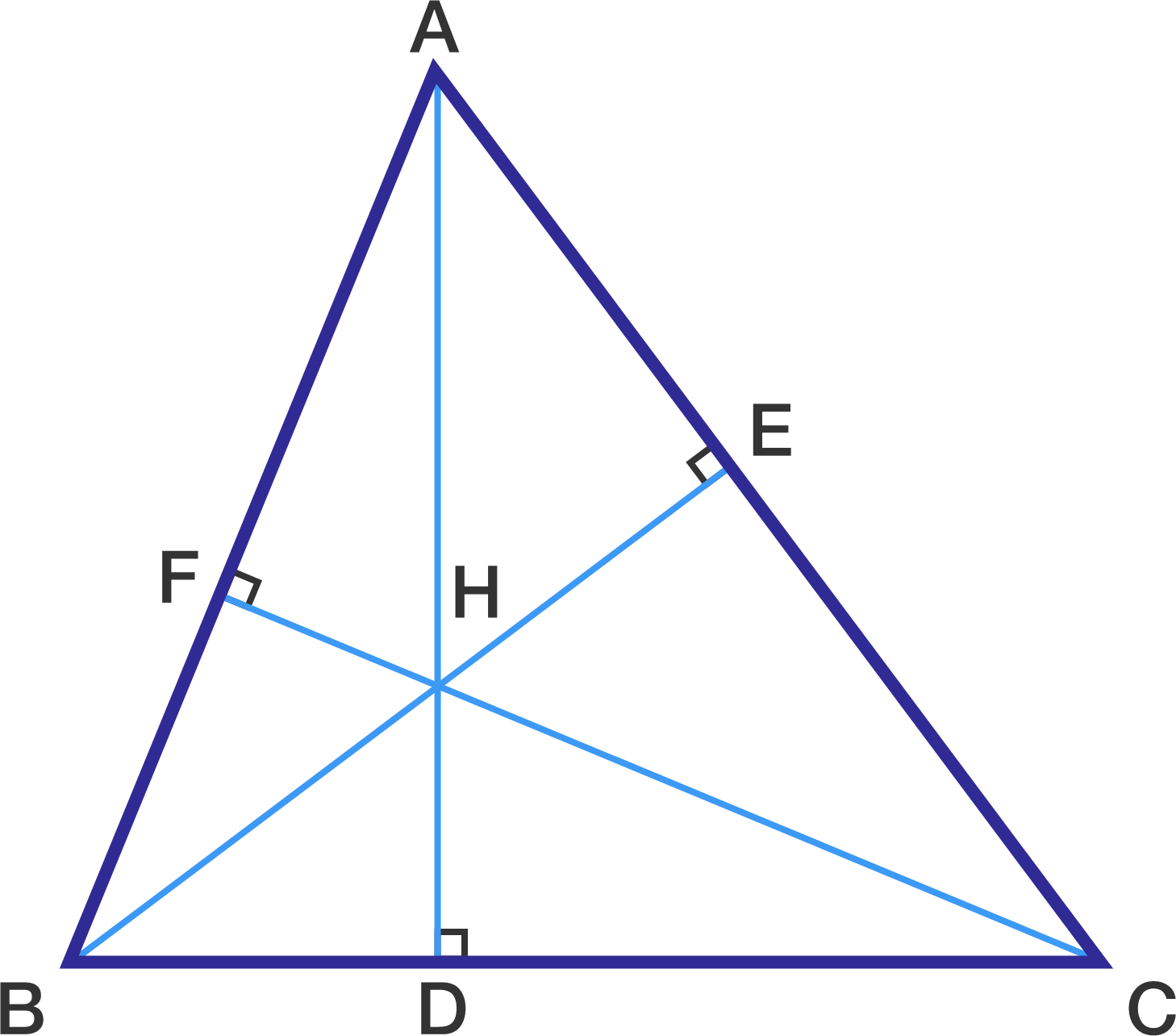# 400 followers problem!

Geometry Level 4Consider $\Delta ABC$ such that $AB = 13, BC = 14, AC = 15$

Let $AD \perp BC, BE \perp AC, CF \perp AB$ and let $H$ be its orthocenter

Let $R(ABC)$ denote circumradius of $\Delta ABC$

Let $r_0$ be inradius and $r_1, r_2, r_3$ be the exradii of $\Delta ABC$

Then find the value of

$R(ABC) + R(HAB) + R(HBC) + R(HAC) + \sum_{i=0}^3 r_i$

×